American Journal of Theoretical and Applied Statistics
Volume 5, Issue 4, July 2016, Pages: 234-241

Extreme Values Modelling of Nairobi Securities Exchange Index

Kelvin Ambrose Kiragu1, Joseph Kyalo Mung’atu2

1Applied Statistics, Department of Statistics and Actuarial Science, Jomo Kenyatta University of Agriculture and Technology, Nairobi, Kenya

2Statistics, Department of Statistics and Actuarial Science, Jomo Kenyatta University of Agriculture and Technology, Nairobi, Kenya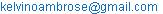(K. A. Kiragu)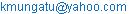(J. K. Mung’atu)

Kelvin Ambrose Kiragu, Joseph Kyalo Mung’atu. Extreme Values Modelling of Nairobi Securities Exchange Index. American Journal of Theoretical and Applied Statistics. Vol. 5, No. 4, 2016, pp. 234-241. doi: 10.11648/j.ajtas.20160504.20

Received: June 21, 2016; Accepted: June 28, 2016; Published: July 13, 2016

Abstract: Extreme events and the clustering of extreme values provide fundamental information which can be used for risk assessment in finance. When applying extreme value analysis to financial time series we handle two major issues, bias and serial dependence. The main objective of the study will be to model the extreme values of the NSE all share index using EVT method thus contributing to empirical evidence of the research into the behavior of the extreme returns of financial series in East Africa and specifically Kenya. This study will model the extreme values of the Nairobi Securities Exchange all share index (2008-2015) by applying the Extreme Value Theory to fit a model to the tails of the daily stock returns data. A GARCH-type model will be fitted to the data to correct for the effects of autocorrelation and conditional heteroscedasticity before the EVT method is applied. The Peak-Over-Threshold approach will be employed with the model parameters obtained by means of Maximum Likelihood Estimation. The models goodness of fit will be assessed graphically using Q-Q and density plots.

Keywords: Extreme Value Theory (EVT), Generalized Pareto Distribution (GPD), Peaks-Over-Threshold (POT), Nairobi Securities Exchange (NSE), NSE All Share Index (NASI)

Contents

1. Introduction

1.1. Background of the Study

Extreme Value Theory (EVT) deals with events that are more extreme or in the probability sense have a low probability i.e. inferences are made beyond the sample data and to do that one would need those observations which are extreme in some sense. However, one should be careful in distinguishing between an outlier and an extreme value. In Extreme Value Theory (EVT), the role of the Normal and the Stable distributions of classical statistics are played by two distributions these are the Generalized Extreme values (GEV) and the Generalized Pareto Distribution (GPD). What makes the EVT very appealing is the fact that the nature of the asymptotic distribution does not necessarily depend on the exact distribution of returns. This study employed secondary data which was obtained from the Nairobi Securities Exchange (NSE). The data consisted of daily closing prices of NSE All Share Index (NASI) spanning the years January 2008 to April 2016. This index is a weighted index based on the values of securities of each of the companies listed on the NSE.

1.2. Statement of the Problem

Despite NSE playing a vital role in contributing to economic prosperity and capital market development in East Africa and specifically Kenya, it faces a number of challenges. Among this challenges include the low market confidence with high level of inflation among others. The issues of concern to most risk managers and financial analysts are the events that occur under extreme market conditions. This is to mean events which have a tendency to produce huge unexpected losses that can lead to bankruptcy. The available body of knowledge focuses more on VaR and expected shortfall as risk measures while studies on NSE have not necessarily addressed EVT methods thus the need to examine the performance of the extreme value methods in the analysis of the stock market with reference to NSE.

1.3. Justification of the Study

EVT-based estimates of VaR directly concentrates on the tails of the distribution, thus avoiding a major flaw of parametric approaches, whose estimates are somehow biased by the credit they give to the central part of the distribution, thus underestimating extremes and outliers, which are exactly what one is interested in when calculating VaR. Also EVT allows one to concentrate on each one of the two tails of the distribution independently, thus allowing a flexible approach which can take skewness (a typical feature of financial time series) of the underlying distribution into account.

1.4. Objectives

1.4.1. General Objective

To model the extreme values of the NSE all share index using Extreme Value Theory.

1.4.2. Specific Objective

1.  To fit an EVT model on NSE all share index.

2.  To construct a risk measure based on the EVT approach.

2. Literature Review

EVT provides a firm theoretical foundation on which we can build statistical models describing extreme events. The foundations of the theory were laid down by Fisher  and Gnedenko , who proved that the distribution of the extreme values of an independent and identically distributed (iid) sample from a cumulative distribution function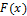, when adequately rescaled, can converge (and indeed does converge, for the majority of known cumulative distribution functions) to one out of only three possible distributions. These limiting distributions are Fréchet, Gumbel and Weibull families. Furthermore, these three types of distributions can be nested into a single class of continuous probability distributions called the GEV distribution given by Hξ (x) Embrechts .

Based on the Pickands-Balkema-de Haan theorem [7-8] the POT model focuses on the distribution of exceedances above some high threshold µ. The interest here is the distribution of the values of x above the threshold µ. According to Nortey  on Ghana stock exchange index they concluded that the daily returns of the Ghana stock exchange all-share index data was from a distribution with fat-tails and asymmetric in nature as a result of the observed volatility in the daily returns data, the conditional EVT approach was preferred for the study. A similar realization was made by Polakow  when they compared the conditional and unconditional approaches in the modelling of a volatile South African stock market, and the conditional approach provided better results compared to the unconditional approach.

Djakovic  concluded that in emerging markets, different characteristics are observed at each of the tails of the return distributions which indicates that risk and reward are not equally likely in these markets. Giles  however concluded that the GPD performs very well in modelling both the positive and negative returns of the tails distributions. It was further indicated by Gencay  that EVT based VaR estimates were more accurate at higher quantiles. Moreover, they reveal that the different daily return distributions have different moment properties at their right and left tails, and as some studies, including Krehbiel  concluded, the upper and lower tails behave differently, and thus should be treated separately while estimating risk measures.

Results of the empirical analysis on Montenegrin stock exchange show that the assessments of Value at Risk based on extreme value theory outperform econometric and quantile evaluations according to Cerovic  concluding that econometric evaluations prove to be on the lower bound possible Value at Risk movements.

Despite the general assertions that financial data specifically stock price data are fat-tailed according to Louangrath  empirical test of data from Thailand’s Stock Exchange shows that the stock market price distribution contains no extreme values under the standard score formula approach for verifying exceedances. Hence suggesting that price volatility index is a better indicator for risk management. Mwamba  found that the Islamic market index exhibits fat tail behavior in its right tail with high likelihood of windfall profit during extreme market conditions probably due to the ban on short selling strategies in Islamic finance. However, the conventional stock markets are found to be more risky than the Islamic markets, and exhibit fatter tail behavior in both left and right tails. Their findings suggest that during extreme market conditions, short selling strategies lead to larger financial losses in the right tail than in the left tails.

3. Research Methodology

3.1. Introduction

The two main methods under EVT approach are the Block Maxima methods and the Peak-Over-Threshold (POT). The distribution of block maxima can be modeled by fitting the GEV to the set of block maxima although according to Ren  this may be an issue whenever one block contains more extremes than another hence the use of a more modern and more powerful tool for modeling extreme events referred to us the peaks-over-threshold (POT) method. POT method will be preferred since it utilizes more of the data thus producing more reliable findings compared to the Block Maxima approach Frey .

3.2. Preliminary Data Analysis

3.2.1. Quantile-Quantile Plot

A popular tool in conducting exploratory data analysis is the Q-Q plot. The QQ-plot is a graphical technique to check whether our sample data is consistent with some known distribution and thus can be used to assess goodness of fitting. A QQ-plot compares the quantiles of the empirical distribution function with the quantiles of the reference distribution model. If the data is from an exponential distribution, the points on the graph would lie along a positively sloped straight line. If there is a concave presence, this would indicate a fat-tailed distribution, whereas a convex departure is an indication of a short-tailed distribution Ren .

3.2.2. The Mean Excess Function

A second useful tool for discrimination in the tail is the mean excess function (MEF) defined by: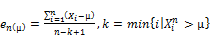(1)

Where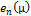is the sample mean excess function,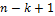is the number of observations exceeding the threshold µ Gencay .

3.3. Threshold Determination

3.3.1. Plot of Mean Excess

The choice of the threshold is critical in order to adopt the POT method to model the tails of the distribution of daily returns and a graphical tool that is very helpful for the selection of the threshold µ is the sample mean excess plot. The sample excess function, which is an estimate of the mean excess function,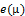is defined as: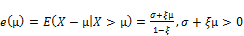(2)

this property is then used as a criterion for the selection of µ.

3.3.2. Hill Plot

Another tool in threshold determination is the Hill-plot. It is based on the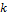upper order statistics of an iid sample. By ordering the data with respect to their values as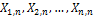where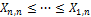the Hill’s estimator of the tail index ξ is(3)

Where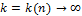is upper order statistics (the number of exceedances), n is the sample size and α=1/ξ is the tail index. A Hill-plot is constructed such that estimated ξ is plotted as a function ofupper order statistics or the threshold. A threshold is selected from the plot where the shape parameter ξ is fairly stable.

3.4. Parameter Estimation

The maximum likelihood method for estimating parameters for a statistical model was used. In this method the probability density function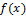can be unknown but the joint density function for the data is assumed to come from a known family of distributions. For an independent and identically distributed sample of size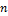the joint density function looks like(4)

where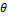are the parameters of the model and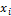are the observed variables. Thus the observed variablesare known whereas the parameters given byare to be estimated. The likelihood function is then given by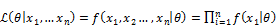(5)

And the often used log-likelihood function is given by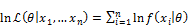(6)

The estimated parameters are then given by the set which maximizes the likelihood function, equation (5) or (6).

3.5. Description of the Data

In this study, the data consisted of daily closing prices of NASI spanning the years January 2008 to May 2016. The reasoning being it represents a measure of the overall performance of the stock market, thus enhancing the possibility of performing accurate estimates of the parameters governing the tail behavior of the distribution. Since financial markets usually only provide the raw data of the realized values of the various financial indices, the daily log-returns will be used interchangeably with returns

4. Results and Discussion

4.1. Introduction

Different packages that deal with extreme in R have been used including fExtremes, rugarch and ismev. This chapter describes how extreme values of the NSE all share index where modeled using extreme value approach.

The yearly progression of the data is illustrated by a logarithmic time series plot as in figure 1. The volatility of the financial returns over the period January 2008 to beginning of May 2016 is examined. It can be seen clearly that the Kenyan stock market experienced some periods of relatively calm and periods of high volatility. Volatility clustering can be observed where periods of high or low changes in the return are accompanied by other high or low changes.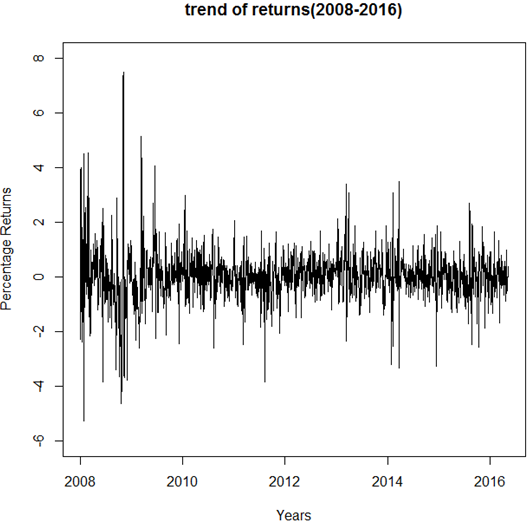Figure 1. Log returns of daily NSE-All Share Index.

Table 1 presents a descriptive statistics of the returns data. The data has a positive mean and a positive skewness value which indicate that the bulk of the data is on the right tail of the distribution of the data. The series also has a high kurtosis value higher than the normal distribution value of 3 which indicates presence of fat tails of the returns data. The Jargue-Bera test for normality showed a p value of 0.0000 hence leading to the rejection of the hypothesis of the data being normally distributed.

Table 1. Descriptive Statistics.

 Description Values Mean 0.020363 Median 0.010492 Maximum 7.485723 Minimum -5.265558 Standard Deviation 0.898864 Skewness 0.662822 Kurtosis 10.196425 Jarque-Bera Test 9145.812 (Probability) 0.0000 Number of Observations 2071

The presumption of financial returns having fat tails was made from an examination of the histogram of returns, figure 2. The histogram shows that relatively more observations lie to the right of the mean of the distribution, compared to the left. This is consistent with the positive skewness value obtained and the high peak corresponding to the large kurtosis value obtained. The curve represents the density curve of the returns. From the graph it can be observed that the density curve has a very high peak around its mean and relatively fatter tails compared to the normal curve. This further confirms that the data deviates from normality.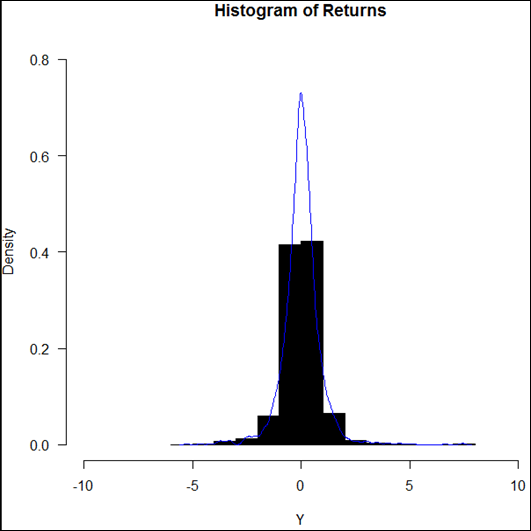Figure 2. Histogram of Returns of daily NSE-All Share Index.

Stationarity was tested by performing Phillips-Perron and Augmented Dickey-Fuller unit root test and it was found that the returns data is fairly stationary since the ρ- value (0.01) < 0.05. It is recommended that the data be iid before applying Extreme Value Method, therefore a Box-Ljung test for autocorrelation was performed. The presence of significant autocorrelation in the return series was present. Further a Lagrangian Multiplier test was performed to test for Autoregressive Conditional Heteroscedastic (ARCH) effects and this reveled presence of significant ARCH effects in the data.

According to McNeil  in order to produce a complete iid process with relatively no autocorrelation and no heteroscedastic effects one would need to fit a GARCH-type model to the data. Therefore, different combinations of ARMA (p, q)-GARCH (m, s) models were fitted and based on BIC and AIC values, the ARMA(1, 1)-GARCH(1, 1) model was found to be the best fitting. The model parameters are shown in Table 2. A standardized iid series was then calculated by dividing the residual terms at time t with the corresponding conditional standard deviation.

Table 2. Results from ARMA-GARCH model.

 Optimal Parameters Estimate Standard Error ρ-value Mu 0.05091 0.02147 0.0177 AR (1) 0.46231 0.05659 0.000 MA (1) -0.12836 0.06434 0.0460 Omega 0.05340 0.01121 0.0000 Alpha 1 0.23761 0.03096 0.0000 Beta 1 0.68603 0.04006 0.0000

No autocorrelation existed also no evidence of volatility clustering in the series and no conditional heteroscedastic terms in the standardized series after performance of autocorrelation and conditional heteroscedasticity tests. The series was therefore considered suitable for application of extreme value analysis.

4.2. Threshold Determination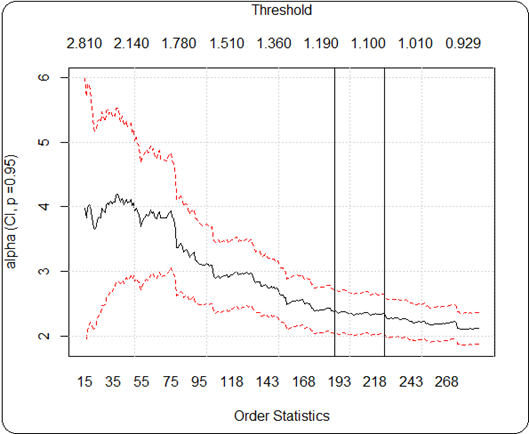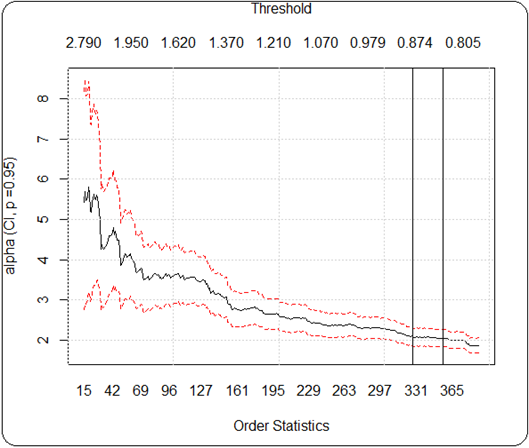Figure 3. Hill plots with right tail on the left and left tail on the right.

The first step under Peak-Over-Threshold approach under EVT is to determine the threshold. This was performed graphically in this study by use of hill plots, shape parameter plots and mean excess plots. The hill plots for the tails of the standardized series are shown in figure 3. The last 290 order statistics are plotted for the right tail and the last 390 for the left tail leaving more than 10% of the data for analysis. The interest with the hill plot was to find a relatively steady area on the plot where the order statistics obtained is sufficiently large and the threshold selected be relatively steady and provide sufficient exceedances to be fitted by the GPD. The area on the right tail plot was determined to be between 189 and 224 order statistics and on the left tail plot the area was determined to be between 327 and 356 indicated by vertical lines in both plots. Therefore a sufficient threshold was expected to lie within these ranges.

The shape parameter plots in figure 4 showed that plots are relatively steady in the ranges determined by the hill plots. These ranges have also been indicated by vertical lines on the plot. The dotted vertical line on the right tail of the shape parameter plot further separates the more steady area within the ranges determined by the hill plot above. The corresponding thresholds determined for the right tail plot were between 1.120-1.165 and for the left tail plot 0.838-0.877.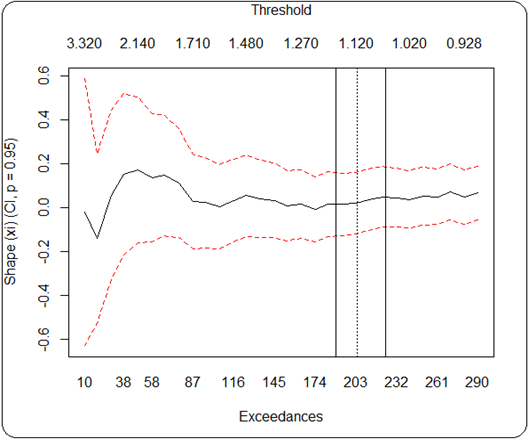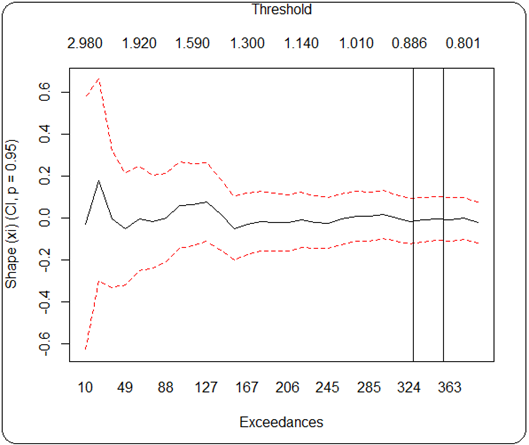Figure 4. Shape Parameter plot with the right tail on the left.

Finally, the mean excess plots were graphed as in figure 5, and based on the hill and shape parameter plots above; the thresholds were expected to lie in a particular range. Upon close examination of the plot of mean excesses, a threshold value of 1.15 for the right tail plot and 0.84 for the left tail plot was determined.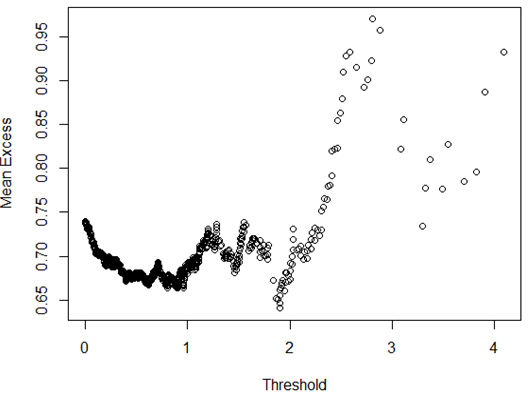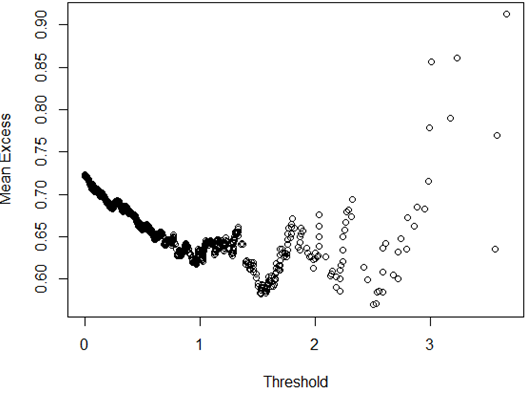Figure 5. Mean Excess plot with the right tail on the left.

It can be noticed that the plots are relatively stable up to the points selected and from there slight variations are observed. It should be noted that this thresholds lie within the ranges determined by the hill plots and shape parameter plots.

4.3. Fitting a Generalized Pareto Distribution

Number of observations above the selected threshold left for modelling on the right tail was 195 and 354 for the left tail. This were more than 10% of the total number of observations in each of the tails thus considered fit for fitting a GPD. It can also be noted that the slopes above the selected threshold have an upward slope thus will be well approximated by the GPD with positive shape parameter.

Table 3 reports the shape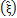and the scaleparameters from the fitted GPD to the tails of the standardized series, and there corresponding standard errors. It was observed that the standard errors of the right tail were greater compared to the left tail. This is an indication that the GPD provided better fit for the left tail compared to the right. There are only positive shape parameters for both the left and right tail this is an indication of the NSE-All Share Index having fatter tails than the normal distribution.

Table 3. Results from the fitted Generalized Pareto Distribution.

 Estimates MLE Right tail µ=1.15 Left tail µ=0.84 Shape Parameter 0.204217 0.048170 Std Error 0.256639 0.113611 Scale Parameter 0.578913 0.578579 Std Error 0.175336 0.088531

4.4. Model Checking

Figure 6 shows plots of the estimated GPD models fitted against the empirical excesses over the selected thresholds. Since all points lie close to the curve of empirical excesses then it can be concluded that the estimated GPD models provide a good fit to the extreme values.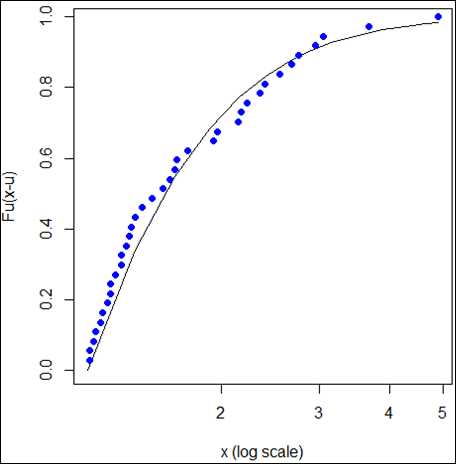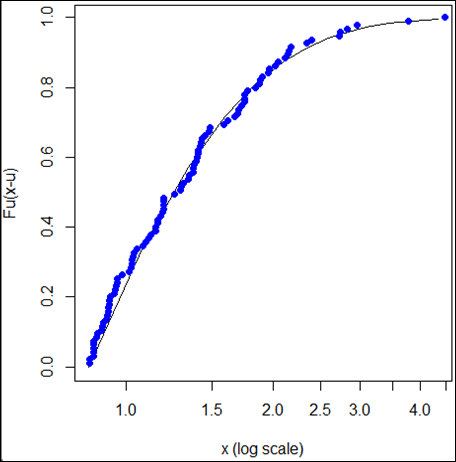Figure 6. Plots for fitted GPD model with the right tail on the left.

Figure 7 provides the plots of the probability for the left and right tail of the goodness of fit of the GPD models on the empirical excesses. Although both models provide good fits, the GPD model of the left tail fits better than the right tail fit. This is because the plot of the right tail indicates more departures from the straight line compared to the left tail plot.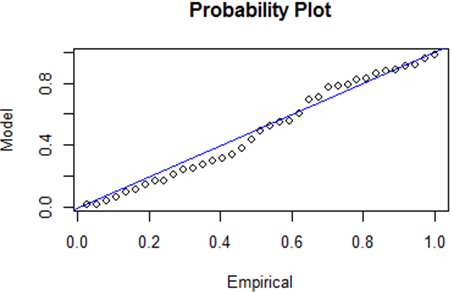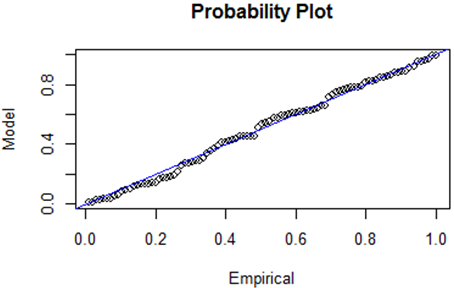Figure 7. Probability plots for the fitted GPD with the right tail on the left.

Q-Q plots are represented on figure 8 the plots show that the points do not deviate significantly from the straight line. The plots of the density in figure 9 verifies the conclusion obtained from the probability plots that the GPD models provide good fits to the exceedances but the model on the left tail provides a better fit since the right tail shows a few departures from the curve.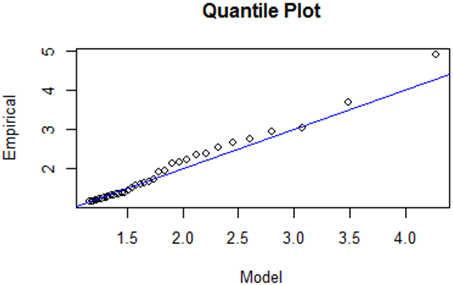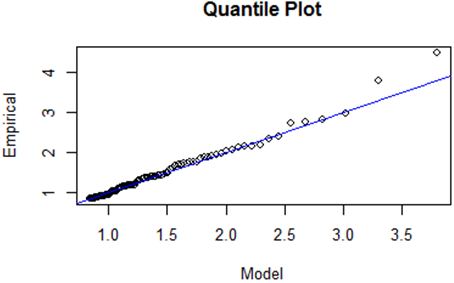Figure 8. Q-Q plot for the fitted GPD model with the right tail on the left.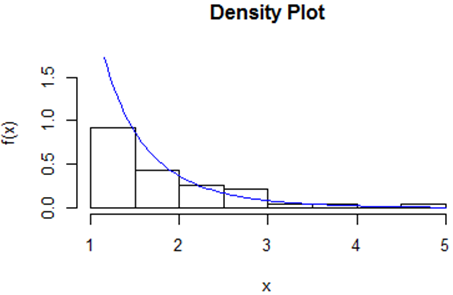Figure 9. Density plots with the right tail on the left.

Return level plots are considered desirable if there are no significant departures from the curve or no points outside the confidence bands represented above and below the curve by blue curves. Figure 10 presents the return level plots for both tails. It can be seen from the plots that no points lay outside of the confidence bands.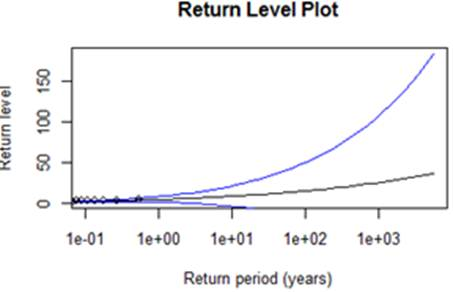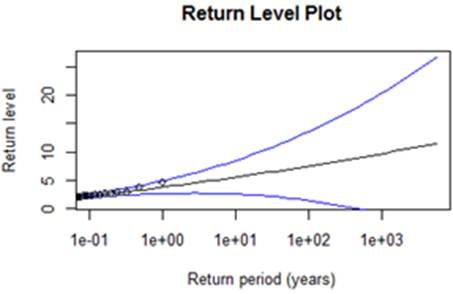Figure 10. Return level plots with the right tail on the left.

4.5. Risk Measure

Risk measures related with the right and left tails after fitting the GPD are presented on Table 4 which is presented in percentages.

Table 4. Risk Measures.

 Probability VaR ES Measure of Risk (right tail) 0.9900 3.485851 4.812761 0.9950 4.272103 5.800784 0.9990 6.590082 8.713611 0.9995 7.848366 10.294801 0.9999 11.557960 14.956364 Measures of Risk (left tail) 0.9900 2.902482 3.614718 0.9950 3.380319 4.116737 0.9990 4.553328 5.349109 0.9995 5.087214 5.910013 0.9999 6.397817 7.286942

The results indicate that with probability of 0.01 hence a 99.0% level of confidence, the expected market return would not gain by more than 3.49% and if it does by any chance increase by more than 3.49% then an average gain of 4.81% is expected within a one day period. If for any reason a loss is experienced then it will not exceed 2.90% with probability 0.01 and the loss if it does exceed 2.90% then the expected loss will be 3.61%.

With probability 0.005 thus a 99.5% level of confidence, the daily market gain will not be more than 4.27% but if it does go beyond then it will not exceed 5.80%. In the case of losses with probability 0.005 the loss will not exceed 3.38% but if it does exceed this then the loss is expected to be 4.12%.

For the higher quantiles with probability 0.0005 that is 99.95% level of confidence the expected market gain is 7.85% and if it does exceed this then it is expected to be 10.29%. On the other hand a loss of 5.09% is expected but if it does exceed this then the expected loss is 5.91%. Additionally, with probability 0.0001, 99.99% level of confidence the expected gain is 11.56% if it exceeds this then it is expected to be 14.96%. A loss is not expected to go beyond 6.40% with probability 0.0001 but if it exceeds this then it is expected to be 7.29%.

These results indicate that for an investment in the NSE, the probability of losses is lower compared to the possibility of gains.

5. Conclusion

This study makes use of the peak-over-threshold method (POT) based on the Generalized Pareto distribution in modelling the tails of the empirical distribution of the NSE-All Share Index. The main objective of the study was to model the extreme values of the NSE-All Share Index using extreme value theory. To achieve this objective the study begun by calculating the logarithmic returns from the daily closing prices of the index. The yearly progression of the data was shown by use of a logarithmic time series plot. Presumption of the data having fat tails was illustrated by a histogram of returns which showed that most of the data lied on the right this was consistent with the high positive value of kurtosis (leptokurtic). The returns data was tested for stationarity and was found to be fairly stationary. The presence of ARCH effects was observed after performing the Lagrangian multiplier test. To produce a complete iid process with no ARCH effects an ARMA (1, 1)-GARCH (1, 1) was fitted to the data. The threshold was determined by use of the Hill plot, Shape parameter plot and the mean excess plot for each of the tails. The right tail was found to be 1.15 and the left tail was 0.84. These thresholds were then used to fit a Generalized Pareto distribution with the parameters shapeand scalebeing estimated by maximum likelihood estimation.

The model was then checked for goodness of fit and it was concluded that both the right tail and left tail fitted the data well but the left tail fitted better compared to the right. Risk measures of VaR and ES were also performed and this indicated that for an investment in the Nairobi Securities Exchange, the probability of losses is lower compared to that of gaining if one was to invest in the NSE.

References

1. R. A. Fisher and L. H. C. Tippett, ‘Limiting forms of the frequency distribution of the largest or smallest member of a sample’, Proceedings of the Cambridge Philosophical Society, 24, 1928, pp. 180-190.
2. B. V. Gnedenko, ‘Sur la distribution limite du terme d’une serie aleatoire’, Annals of Mathematics, 44, 1943, pp. 423-453.
3. P. Embrechts, C. Kluppelberg and T. Mikosch, ‘Modelling extremal events for insurance and finance’, Springer-Verlag, Berlin, 1997.
4. D. M. Mason, ‘Laws of large numbers for sums of extreme values’, Annals of Probability, Vol 10, No 3, 1982, pp. 754-764.
5. F. Ren and D. E. Giles, ‘Extreme value analysis of daily Canadian crude oil prices’, Econometrics Working Paper, EWP 0708. University of Victoria, 2007.
6. A. J. McNeil and R. Frey, ‘Estimation of tail-related risk measures for heteroscedastic financial time series: an extreme value approach’, Journal of Empirical Finance 7, 2000, pp. 271-300.
7. J. Pickands, ‘Statistical inference using extreme order statistics’, Annals of Statistics, 3, 1975, pp. 119-131.
8. A. Balkema and L. deHaan, ‘Residual lifetime at great age’, Annals of Probability, 2, 1974, pp. 792-804.
9. E. N. Nortey, K. Asare and O. Mettle, ‘Extreme value modelling of Ghana stock exchange index’, Springer plus 2015, Department of statistics, University of Ghana.
10. D. A. Polakow and A. J. Seymour, ‘A coupling of extreme-value theory and volatility updating with value-at-risk estimation in emerging markets’, South African test. Multinational Finance Journal, 7, 2003, pp. 3-23.
11. V. Djakovic, G. Andjelic and J. Borocki, ‘Performance of extreme value theory in emerging markets: An empirical treatment’, African Journal of Business Management, Vol 5 (2), 2011, pp. 340-369.
12. G. Bi, & D. Giles, ‘An application of extreme value analysis to U .S. movie box office returns’, Econometrics Working Paper EWP0705, 2007.
13. R. Gencay and F. Selcuk, ‘Extreme value theory and value-at-risk: Relative performance in emerging markets’, International Journal of Forecasting, Vol 20 (2), 2004, pp. 287-303.
14. T. Krehbiel and L. C. Adkins, ‘Price risk in the nymex energy complex: An extreme value approach’, Journal of Future Mark, 25, 2005, pp. 309-337.
15. J. Cerovic, M. Lipovina-Bozovic and S. Vujosevic, ‘A comparative analysis of value at risk measurement on emerging stock markets: Montenegro case’, Business Systems Research Journal, Vol 6 (1), 2015, pp. 36-55.
16. P. I. Louangrath, ‘Stock price analysis under extreme value theory’, Bangkok University International College, Thailand, 2016.
17. J. W. M. Mwamba, S. Hammoudeh and R. Gupta, ‘Financial tail risks and the shapes of the extreme value distribution: A comparison between conventional and sharia-compliant stock indexes’, University of Pretoria Department of Economics Working Paper Series No. 201480, 2014.
18. A. J. McNeil and R. Frey, ‘Estimation of Tail-related Risk Measures for Heteroscedastic Financial Time Series: an extreme value approach’, Journal of Empirical Finance 7, 2000, pp. 271-300.

 Contents 1. 1.1. 1.2. 1.3. 1.4. 2. 3. 3.1. 3.2. 3.3. 3.4. 3.5. 4. 4.1. 4.2. 4.3. 4.4. 4.5. 5.
Article ToolsAbstractPDF(1838K)